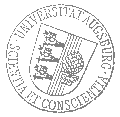# Universität AugsburgInstitut für MathematikOberseminar Stochastik

University of Warwick

spricht am

Freitag, 21. Juli 2023

um

16:00 Uhr

im

Raum 2004 (L1)

über das Thema:

## » Scaling limits for non-convex interaction«

 Abstract: We introduce a currently hot topic in probability theory, the theory of scaling limits for random fields of gradients in all dimensions. The random fields are a class of model systems arising in the studies of random interfaces, random geometry, Euclidean field theory, the theory of regularity structures, and elasticity theory. After explaining how non-convex energy terms can influence the scaling limit, we outline our result on the scaling limit to the continuum Gaussian Free Field in dimension d=2,3 for a class of non-convex interaction energies. We show that the Hessian of the free energy governs the continuum Gaussian Free Field. The second result concern the Gaussian decay of correlations. All our results hold in the low-temperature regime and moderate boundary tilts. We outline how multi-scale/renormalisation group methods provide means of proving our statements. if time permits we discuss isomorphism theorems for the model.

 Hierzu ergeht herzliche Einladung. Prof. Dr. Markus Heydenreich

[Impressum]      [Datenschutz]      wwwadm@math.uni-augsburg.de,     Di 11-Jul-2023 06:46:53 MESZ